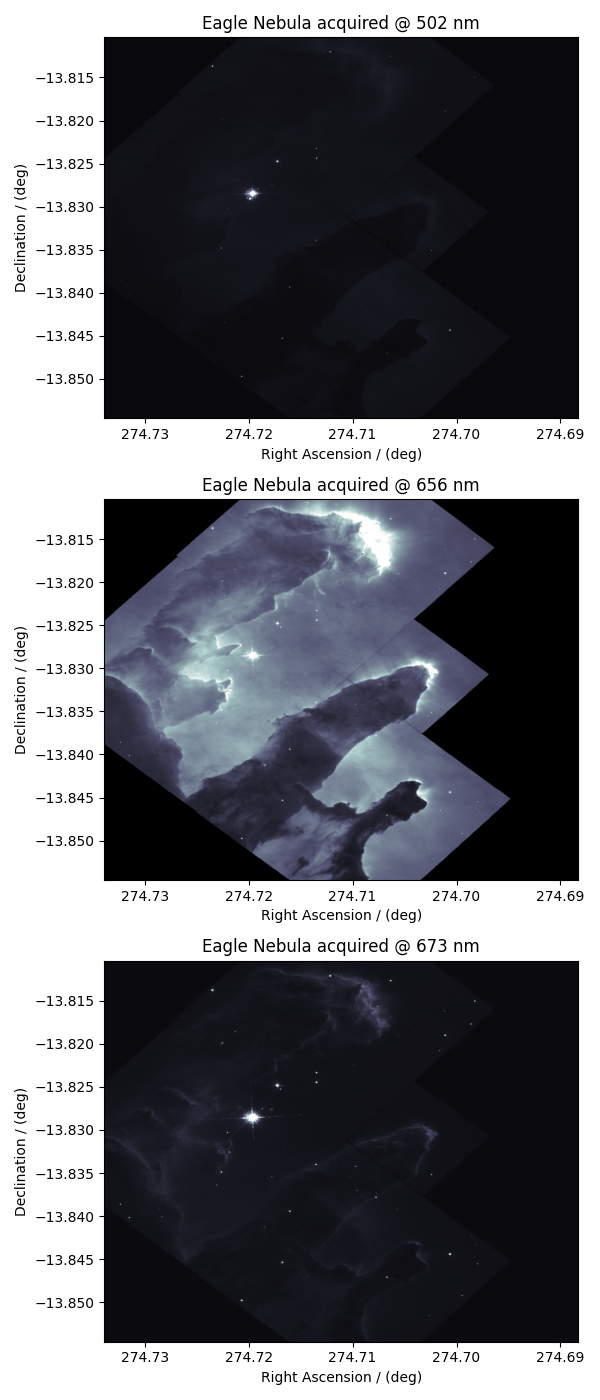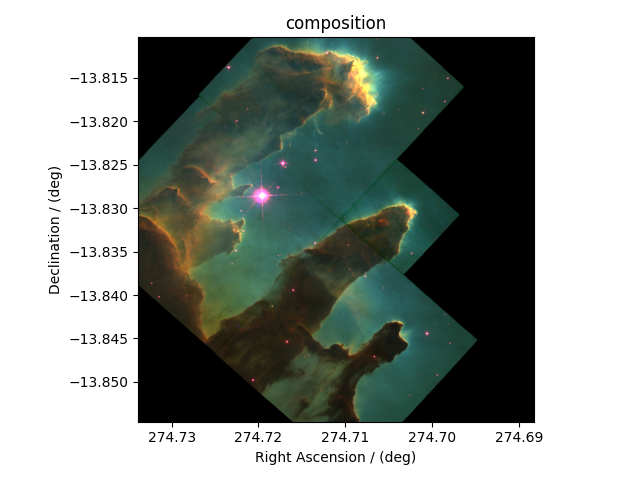Astronomy, 2D{1,1,1} dataset (Creating image composition)¶

More often, the images in astronomy are a composition of datasets measured at different wavelengths over an area of the sky. In this example, we illustrate the use of the CSDM file-format, and csdmpy module, beyond just reading a CSDM-compliant file. We’ll use these datasets, and compose an image, using Numpy arrays. The following example is the data from the Eagle Nebula acquired at three different wavelengths and serialized as a CSDM compliant file.

Import the csdmpy model and load the dataset.

import csdmpy as cp

filename = "https://osu.box.com/shared/static/of3wmoxcqungkp6ndbplnbxtgu6jaahh.csdf"

Let’s get the tuple of dimension and dependent variable objects from the eagle_nebula instance.

Before we compose an image, let’s take a look at the individual dependent variables from the dataset. The three dependent variables correspond to signal acquisition at 502 nm, 656 nm, and 673 nm, respectively. This information is also listed in the name attribute of the respective dependent variable instances,

print(y.name)

Out:

Eagle Nebula acquired @ 502 nm
print(y.name)

Out:

Eagle Nebula acquired @ 656 nm
print(y.name)

Out:

Eagle Nebula acquired @ 673 nm

Data Visualization¶

For convince, let’s view this CSDM object with three dependent-variables as three CSDM objects, each with a single dependent variable. We use the split() method.

Here, data0, data1, and data2 contain the dependent-variable at index 0, 1, 2 of the eagle_nebula object. Let’s plot the data from these dependent variables.

import matplotlib.pyplot as plt

_, ax = plt.subplots(3, 1, figsize=(6, 14), subplot_kw={"projection": "csdm"})

ax.imshow(data0 / data0.max(), cmap="bone", vmax=0.1, aspect="auto")
ax.imshow(data1 / data1.max(), cmap="bone", vmax=0.1, aspect="auto")
ax.imshow(data2 / data1.max(), cmap="bone", vmax=0.1, aspect="auto")

plt.tight_layout()
plt.show()Image composition¶

import numpy as np

For the image composition, we assign the dependent variable at index zero as the blue channel, index one as the green channel, and index two as the red channel of an RGB image. Start with creating an empty array to hold the RGB dataset.

shape = y.components.shape + (3,)
image = np.empty(shape, dtype=np.float64)

Here, image is the variable we use for storing the composition. Add the respective dependent variables to the designated color channel in the image array,

image[..., 0] = y.components / y.components.max()  # red channel
image[..., 1] = y.components / y.components.max()  # green channel
image[..., 2] = y.components / y.components.max()  # blue channel

Following the intensity plot of the individual dependent variables, see the above figures, it is evident that the component intensity from y and, therefore, the green channel dominates the other two. If we plot the image data, the image will be saturated with green intensity. To attain a color-balanced image, we arbitrarily scale the intensities from the three channels. You may choose any scaling factor. Each scaling factor will produce a different composition. In this example, we use the following,

image[..., 0] = np.clip(image[..., 0] * 65.0, 0, 1)  # red channel
image[..., 1] = np.clip(image[..., 1] * 7.50, 0, 1)  # green channel
image[..., 2] = np.clip(image[..., 2] * 75.0, 0, 1)  # blue channel

Now to plot this composition.

# Set the extents of the image plot.
extent = [
x.coordinates.value,
x.coordinates[-1].value,
x.coordinates.value,
x.coordinates[-1].value,
]